# 苯酚含量测定中KI最佳用量的研究Study on the Optimal Dosage of KI in the Determination of Phenol

• 全文下载: PDF(1569KB)    PP.351-357   DOI: 10.12677/HJCET.2018.86046
• 下载量: 250  浏览量: 397

The influence of several factors on the determination of phenol was studied by titration method. The optimum dosage of 6 mol•L−1 HCl is 10 mL, KI is 0.8 g, and the reaction time is 7.5 min. The concentration of phenol is close to the standard value, and the relative error is less than 0.94%. When the dosage of KI is 1.2 g and the dark reaction time is 10 min, the best dosage of KBrO3-KBr can be selected at 8 mL.

1. 引言

2. 实验 

2.1. 原料与试剂

2.2. 实验方法——滴定法 

1) 样品中苯酚含量的测定

${\text{KBrO}}_{\text{3}}+\text{5KBr}+\text{6HCl}={\text{3Br}}_{\text{2}}+{\text{3H}}_{\text{2}}\text{O}+\text{6KCl}$

${\text{C}}_{\text{6}}{\text{H}}_{\text{5}}\text{OH}+{\text{3Br}}_{\text{2}}={\text{C}}_{\text{6}}{\text{H}}_{\text{2}}{\text{OHBr}}_{\text{3}}+\text{3HBr}$

${\text{Br}}_{\text{2}}\left(过量的\right)+\text{2KI}={\text{I}}_{\text{2}}+\text{2KBr}$

${\text{I}}_{\text{2}}+{\text{2Na}}_{\text{2}}{\text{S}}_{\text{2}}{\text{O}}_{\text{3}}=\text{2NaI}+{\text{Na}}_{\text{2}}{\text{S}}_{\text{4}}{\text{O}}_{\text{6}}$

2) 空白实验

3. 结果与讨论Table 1. Equivalence relation and dosage of reagents in the existing methods

3.1. KI的加入量对苯酚浓度测定的影响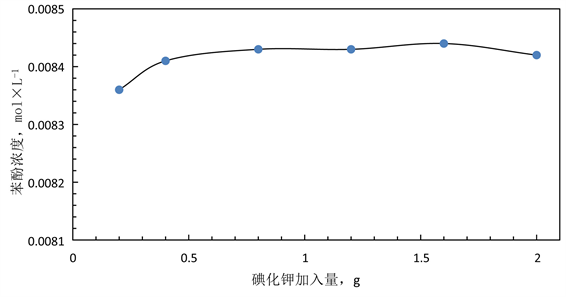Figure 1. Influence of different potassium iodide amounts on phenol concentration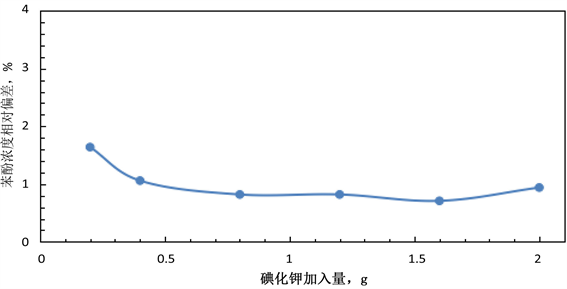Figure 2. The effect of different potassium iodide amounts on the relative deviation of phenol concentration

3.2. 不同暗置反应时间对苯酚浓度测定的影响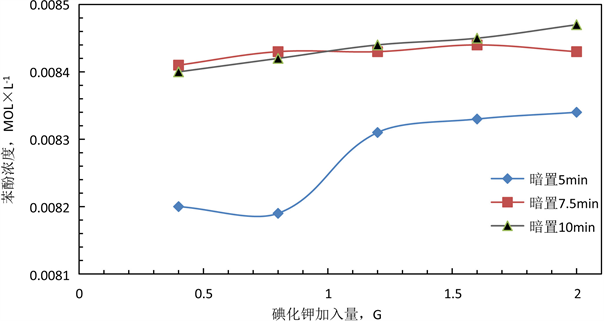Figure 3. Influence of darkening reaction time on determination of phenol concentrationFigure 4. Influence of dark reaction time on relative variation of phenol concentration

3.3. KBrO3-KBr溶液的不同用量对苯酚含量测定的影响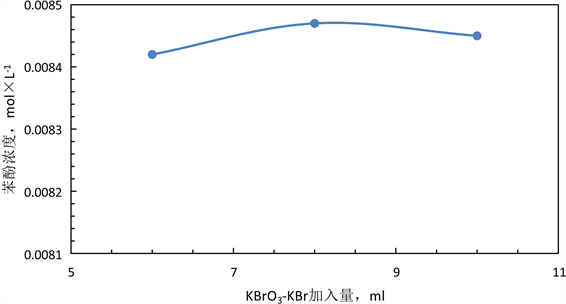Figure 5. The effect of the amount of KBrO3-KBr on the determination of phenol concentration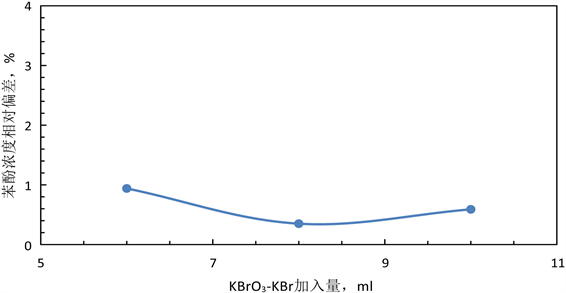Figure 6. The effect of the amount of KBrO3-KBr on the relative deviation of phenol concentration

4. 结论

NOTES

*通讯作者。

  吴肇亮, 俞英, 等, 编. 基础化学实验(上册) [M]. 北京: 石油工业出版社, 2003: 225-228.  俞斌. 无机与分析化学实验[M]. 北京: 化学工业出版社, 2009: 142-144.  俞斌, 吴文源. 无机与分析化学实验[M]. 北京: 化学工业出版社, 2013: 166-169.  国家技术监督局. GB/T14074.13-1993木材胶粘剂及其树脂检验方法游离苯酚含量测定方法[S]. 1993-03-18.  国家药典委员会, 编. 中华人民共和国药典[M]. 北京: 化学工业出版社, 2005: 二部, 325.  陈明秀. 酚醛树脂游离酚含量的测定方法[J]. 燃料与化工, 2007, 38(5): 30-32.  张苏静, 黄仁和. 热塑性酚醛树脂合成中废水游离酚含量的测定方法[J]. 铸造, 2013, 62(1): 41-44.  展海军, 李建伟. 无机与分析化学实验[M]. 北京: 化学工业出版社, 2012: 138-140.  务宗伟, 陈合刚. 应用光度法和溴化滴定法测定废水中挥发性酚的比较[J]. 黑龙江环境通报, 2000, 24(3): 68-69, 39.  宋艳茹, 黄玮. 液体稳定剂中游离苯酚的测定[J]. 塑料助剂, 2013(4): 49-50.  郁兰. 工业废水中苯酚的含量测定及色谱分析[J]. 黑龙江科技信息, 2009(33): 63.  国家环境保护总局《水和废水监测分析方法》编委会. 水和废水监测分析方法[M]. 第四版. 北京: 中国环境科学出版社, 2012: 464.